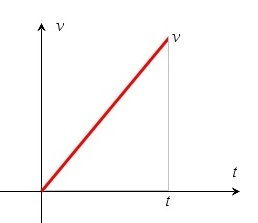# Formulas for the displacement, starting at zero velocity

Up a level : Pre DP, Mechanics and Electronics
Previous page : Linear acceleration from 0
Next page : Formulas for the displacement, starting at non zero velocityStarting at v=0 m/s

Let us get a general formula for the displacement (and distance) traveled as an object accelerate with a constant acceleration from 0 m/s over some time t to a velocity v as described in the figureSince the area under the curve = the area of a triangle we get that the displacement is$s = \frac{{vt}}{2}$

where v is the end velocity. This is by the way an useful formula all by itself. But we will soon get a more general one of a similar type. Anyhow, since v=at for an uniform acceleration from 0, we  may replace v by at in the formula for the displacement to get$s = \frac{{vt}}{2} = \frac{{at \cdot t}}{2} = \frac{{a{t^2}}}{2}$

We thus have that$s = \frac{{a{t^2}}}{2}$

for an uniform, i.e. constant, acceleration from 0 over the time t.

Example : Say an object start at 0 m/s and accelerate at a constant rate of  10 m/s2 over a period of 5 s. How fast is it moving and far has it moved?

We get v=at=10·5=50 m/s and thus s=vt/2=50·5/2=25·5=125 m. We could also calculate this as s=at2/2=10·52/2=10·25/2=5·25=125 m.

Free fall

Dropping a somewhat dense object would, at least initially, accelerate with about 9.8 m/s2. A bit more near the poles, a bit less near the equator. The average is about 9.81 m/s2. This if we assume we have no air friction. A truly frictionless fall is called a free fall. For low enough speeds, and heavy and dense enough object we may count a fall as a free fall.

So  how fast and far would a very heavy and dense object fall over the course of 5 seconds? We calculate the velocity as v=9.81t and the distance as s=9.81t2/2 .

 t [s]: v [m/s]: s [m]: Δs [m]: 0 0.0 0.0 1 9.8 4.9 4.9 2 19.6 19.6 14.7 3 29.4 44.1 24.5 4 39.2 78.5 34.3 5 49.1 122.6 44.1

As we can see we get quite close to the 125 m that we got for 10 m/s2 . It is slightly less since 9.81 m/s2 is a slightly smaller acceleration than  10 m/s2 .

The last  row shows us how far it has travelled over the last second, and as you can see it is comparable to the velocity in the beginning and the end of the interval. It is actually the same as the average velocity times the time over the interval, and why that is, we will show a few sections further on.

A short comment on accuracy

In the table the displacement at say 5 s is reported as 122.6 m. This would require that we would need to know the time as 5.000 s and gravity  need to be know that well too.  Say we know the time as 5.0 s,  so that the actual time is between 4.95 s and 5.05 s, then,  given that a=g=9.81 m/s2, the smallest possible displacement would be, using the shortest time

smin=9.81·4.952/2=120 m

and the biggest possible displacement is

smax=9.81·5.052/2=125 mUp a level : Pre DP, Mechanics and Electronics
Next page : Formulas for the displacement, starting at non zero velocityLast modified: Jan 31, 2017 @ 21:27